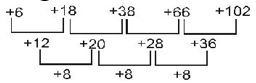# Number Series Questions

Q:

What will be the next term in

BKS, DJT, FIU, HHV, __

 A) IJX B) IGX C) JGW D) JGU

Explanation:

Here the first letter of the group is moved with a gap of one letter, the second letter of the group is backwardness and the third letter of the group is forwardness proceeding like this we get the letter group "JGW"

38 24187
Q:

What is the next number of the following sequence ?

21, 77, 165, 285, ......

 A) 404 B) 415 C) 426 D) 437

Explanation:

21 =
77 = $92-4$
165 = $132-4$
285 = $172-4$

5,9,13,17,21,(+4)...
In the same way next no. will be
$212-4$ = 441 - 4 = 437

39 24051
Q:

Which fraction comes next in the sequence

 A) 1/27 B) 11/278 C) 9/48 D) 7/123

Explanation:

Clearly, the numerators of the fractions in the given sequence follow the series of 2, 3, 5, 7,... and the denominator follows 3, 9, 27, 81, 243, ...
Then, the next term in the given sequence is   .

27 23954
Q:

Find the missing number in the given series ?

15, 51, 216, 1100, ?, 46452

 A) 5660 B) 6560 C) 6750 D) 6630

Explanation:

Let first no. be "k" and multiple be "l"...start value of y with 3
then,
[(k x y) + {l x (l-1)}]
Thus, we get

15 x 3 + 3 x 2 = 51
51 x 4 + 4 x 3 = 216
216 x 5 + 5 x 4 = 1100
1100 x 6 + 6 x 5 = 6630
6630 x 7 + 7 x 6 = 46452

Therefore, the missing number is 6630.

52 23696
Q:

What will come in place of question mark(?) in the following number series ?

6859       5832     ?      4096     3375

 A) 4589 B) 4913 C) 5013 D) 5169

Explanation:

The given series follows a pattern that,

19 x 19 x 19 = 6859

18 x 18 x 18 = 5832

17 x 17 x 17 = 4913

16 x 16 x 16 = 4096

15 x 15 x 15 = 3375

So, the missing number in the given series = 4913

65 22287
Q:

Find the missing number in the given number series?

625, 625, 600, ?, 475, 875

 A) 545 B) 700 C) 675 D) 725

Explanation:

Here the given number series 625, 625, 600, ?, 475, 875 follows a pattern that

625

625 + (0 x 0) = 625

625 - (5 x 5) = 625 - 25 = 600

600 + (10 x 10) = 600 + 100 = 700

700 - (15 x 15) = 700 - 225 = 475

475 + (20 x 20) = 475 + 400 = 875

Hence, the missing number in the given number series is 700.

11 22063
Q:

H T 6 # E 7 $K I L % 3 P @ 2 A J R U 4 * V D What will come in place of the question mark(?) in the following series based on the above arrangement ? T#6 7K$  L3% ?

 A) @2A B) A@2 C) 2A@ D) None of these

Explanation:

20 21414
Q:

Complete the number series given below ?

13        19       37      ?         141        243

 A) 58 B) 67 C) 75 D) 96

Explanation:

The given series follows the pattern thatSo the missing number is  37 + 38 = 75

75 + 66 = 141

....